# NCERT Solutions for Class 7 Maths Chapter 11 Perimeter and Area Ex 11.3

NCERT Solutions for Class 7 Maths Chapter 11 Perimeter and Area Exercise 11.3
Ex 11.3 Class 7 Maths Question 1.
Find the circumference of the circles with the following radius. (Take π =$$\frac{22}{7}$$)
(a) 14 cm
(b) 28 mm
(c) 21 cm
Solution:
(a) Given: Radius (r) = 14 cm
∴ Circumference = 2πr = 2 × $$\frac{22}{7}$$ × 14
= 88 cm
(b) Given: Radius (r) = 28 mm
∴ Circumference = 2πr = 2 × $$\frac{22}{7}$$ × 28
= 176 mm
(c) Given: Radius (r) = 21 cm
∴ Circumference = 2πr = 2 × $$\frac{22}{7}$$ × 21
= 132 cm

Ex 11.3 Class 7 Maths Question 2.
Find the area of the following circles, given that (Take π =$$\frac{22}{7}$$)
(b) diameter = 49 m
Solution:
(a) Here, r = 14 mm
∴ Area of the circle = πr2
= π × 14 × 14 = $$\frac{22}{7}$$ × 14 × 14
(b) Here, diameter = 49 m 49(c) Here, radius = 5 cm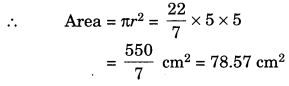Ex 11.3 Class 7 Maths Question 3.
If the circumference of a circular sheet is 154 m, find its radius. Also find the area of the sheet. (Take π =$$\frac{22}{7}$$)
Solution:
Given: Circumference = 154 m
∴ 2πr = 154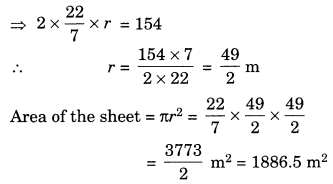Ex 11.3 Class 7 Maths Question 4.
A gardener wants to fence a circular garden of diameter 21 m. Find the length of the rope he needs to purchase, if he makes 2 rounds offence. Also find the cost of the rope, if it costs ₹ 4 per metre. (Take π =$$\frac{22}{7}$$)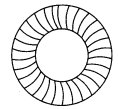Solution:
Diameter of the circular garden = 21 m
∴ Radius = $$\frac{21}{2}$$ m
∴ Circumference = 2πr = $$2 \times \frac{22}{7} \times \frac{21}{2}$$
= 66 m
Length of rope needed for 2 rounds
= 2 × 66 m = 132 m
Cost of the rope = ₹4 × 132 = ₹ 528

Ex 11.3 Class 7 Maths Question 5.
From a circular sheet of radius 4 cm, a circle of radius 3 cm is removed. Find the area of the remaining sheet. (Take π = 3.14)
Solution:
Radius of the circular sheet = 4 cm
∴ Area = πr2 = π × 4 × 4 = 16π cm2
Radius of the circle to be removed = 3 cm
∴ Area of sheet removed = πr2 = 9π cm2
Area of the remaining sheet
= (16π – 9π) cm2 = 7π cm2
= 7 × 3.14 cm2 = 21.98 cm2
Hence, the required area = 21.98 cm2.

Ex 11.3 Class 7 Maths Question 6.
Saima wants to put a lace on the edge of a circular table cover of diameter 1.5 m. Find the length of the lace required and also find its cost if one metre of the lace costs ₹ 15. (Take π = 3.14)
Solution:
Diameter of the table cover = 1.5 m
∴ Radius = $$\frac{1.5}{2}$$ = 0.75 m
∴ Length of the lace = 2πr = 2 × 3.14 × 0.75
= 4.710 m
Cost of the lace = ₹ 15 × 4.710 = ₹ 70.65

Ex 11.3 Class 7 Maths Question 7.
Find the perimeter of the given figure, which is a semicircle including its diameter.Solution:
Given: Diameter = 10 cm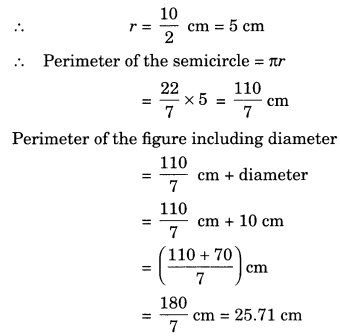Hence, the required perimeter
= 25.7 cm. (approx.)

Ex 11.3 Class 7 Maths Question 8.
Find the cost of polishing a circular table-top of diameter 1.6 m, if the rate of polishing is ₹ 15 m2. (Take π = 3.14)
Solution:
Given:
Diameter = 1.6 m
∴ Radius = $$\frac{1.6}{2}$$ = 0.8 m
Area of the table-top = πr2
= 3.14 × 0.8 × 0.8 m2
= 2.0096 m2
∴ Cost of polishing = ₹ 15 × 2.0096
= ₹ 30.14 (approx.)

Ex 11.3 Class 7 Maths Question 9.
Shazli took a wire of length 44 cm and bent it into the shape of a circle. Find the radius of that circle. Also find its area. If the same wire is bent into the shape of a square, what will be the length of each of its sides? Which figure encloses more area, the circle or the square? (Take π =$$\frac{22}{7}$$)
Solution:
Length of the wire to be bent into a circle = 44 cm
2πr = 44Now, the length of the wire is bent into a square.
Here perimeter of square
= Circumference of line k
Length of each side of the square
$$=\frac{\text { Perimeter }}{4}=\frac{44}{4}=11 \mathrm{cm}$$
Area of the square = (Side)2 = (11)2 = 121 cm2
Since, 154 cm2 >121 cm2
Thus, the circle encloses more area.

Ex 11.3 Class 7 Maths Question 10.
From a circular card sheet of radius 14 cm, two circles of radius 3.5 cm and a rectangle of length 3 cm and breadth 1 cm are removed, (as shown in the given figure below). Find the area of the remaining sheet. (Take π = $$\frac{22}{7}$$)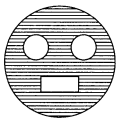Solution:
Radius of the circular sheet = 14 cm
∴ Area = πr2 = $$\frac{22}{7}$$ × 14 × 14 cm2
= 616 cm2
Area of 2 small circles = 2 × πr2
= 2 × $$\frac{22}{7}$$ × 3.5 × 3.5 cm2
= 77.0 cm2
Area of the rectangle = l × b
= 3 × 1 cm2 = 3 cm2
Area of the remaining sheet after removing the 2 circles and 1 rectangle
= 616 cm2 – (77 + 3) cm2
= 616 cm2 – 80 cm2 = 536 cm2

Ex 11.3 Class 7 Maths Question 11.
A circle of radius 2 cm is cut out from a square piece of an aluminium sheet of side 6 cm. What is the area of the left over aluminium sheet? (Take π = 3.14)
Solution:
Side os the square sheet = 6 m
∴ Area of the sheet = (Side)2 = (6)2 = 36 cm2
Radius of the circle = 2 cm
∴ Area of the circle to be cut out = πr2
= $$=\frac{22}{7} \times 2 \times 2=\frac{88}{7} \mathrm{cm}^{2}$$
Area of the left over sheet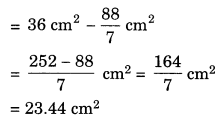Ex 11.3 Class 7 Maths Question 12.
The circumference of a circle is 31.4 cm. Find the radius and the area of the circle. (Take π = 3.14)
Solution:
Circumference of the circle = 31.4 cm
2πr = 31.4
∴ $$r=\frac{31.4}{2 \times 3.14}$$ = 5cm
Area of the circle = 7πr2 = 3.14 × 5 × 5 = 78.5 cm2
Hence, the required radius = 5 cm and area = 78.5 cm2.

Ex 11.3 Class 7 Maths Question 13.
A circular flower bed is surrounded by a path 4 m wide. The diameter of the flower bed is 66 m. What is the area of this path? (Take π = 3.14)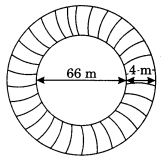Solution:
Diameter of the flower bed = 66 m .
∴ Radius = $$\frac{66}{2}$$ = 33 m
Let r1 = 33 m
Width of the path = 4 m
Radius of the flower bed included path
= 33 m + 4 m = 37m
Let r2 = 37m
Area of the circular path = $$\pi\left(r_{2}^{2}-r_{1}^{2}\right)$$
= 3.14 (372– 332)
= 3.14 × (37 + 33) (37 – 33) [Y a2 – b2 = (a + b)(a-b)]
= 3.14 × 70 × 4 = 879.20 m2
Hence, the required area = 879.20 m2

Ex 11.3 Class 7 Maths Question 14.
A circular flower garden has an area of 314 m2. A sprinkler at the centre of the garden can cover an area that has a radius of 12 m. Will the sprinkler can water the entire garden?
[Take π = 3.14]
Solution:
Area of the flower garden = 314 m2
Radius of the circular portion covered by the sprinkler = 12 m
∴ Area = 7πr2 = 3.14 × 12 × 12
= 3.14 × 144 m2 = 452.16 m2
Since 452.16 m2 > 314 m2
Yes, the sprinkler will water the entire garden.

Ex 11.3 Class 7 Maths Question 15.
Find the circumference of the inner and the outer circles, shown in the given figure. (Take π = 3.14)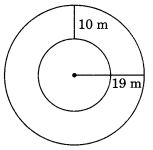Solution:
Radius of the outer circle = 19 m
∴ Circumference of the outer circle = 2πr
= 2 × 3.14 × 19 = 3.14 × 38 m
= 119.32 m
= 19m – 10m = 9m
∴ Circumference = 2πr = 2 × 3.14 × 9
= 56.52 m
Here the required circumferences are 56.52 m and 119.32 m.

Ex 11.3 Class 7 Maths Question 16.
How many times a wheel of radius 28 cm must rotate to go 352 m? (Take π = $$\frac{22}{7}$$)
Solution:
Radius of the wheel = 28 cm
∴ Circumference = 2πr = 2 × $$\frac{22}{7}$$ × 28 = 176 cm
Number of rotations made by the wheel in going 352 m or 35200 cm
$$=\frac{35200}{176}=200$$
Hence, the required number of rotation = 200.

Ex 11.3 Class 7 Maths Question 17.
The minute hand of a circular clock is 15 cm long. How far does the tip of the minute hand move in 1 hour? (Take π = 3.14)
Solution:
Length of minute hand = 15 cm
Circumference = 2πr
= 2 × 3.14 × 15 cm = 94.2 cm
Since the minute hand covers the distance in 1 hour equal to the circumference of the circle. Here the required distance covered by the minute hand = 94.2 cm.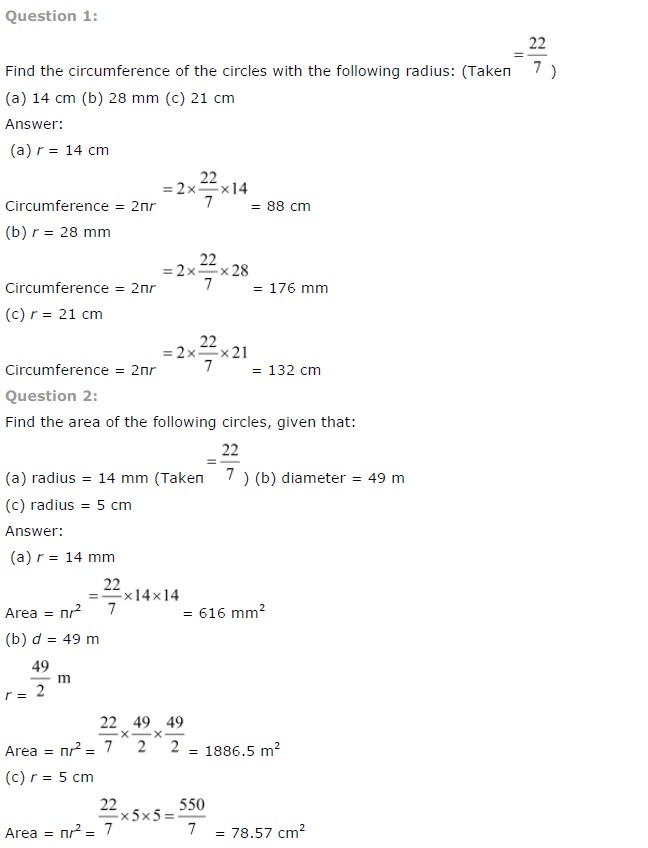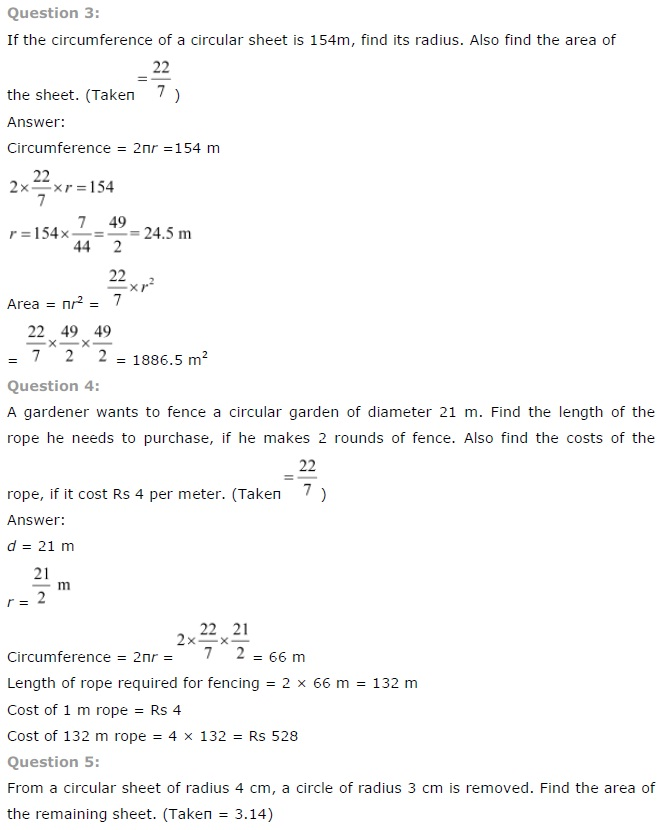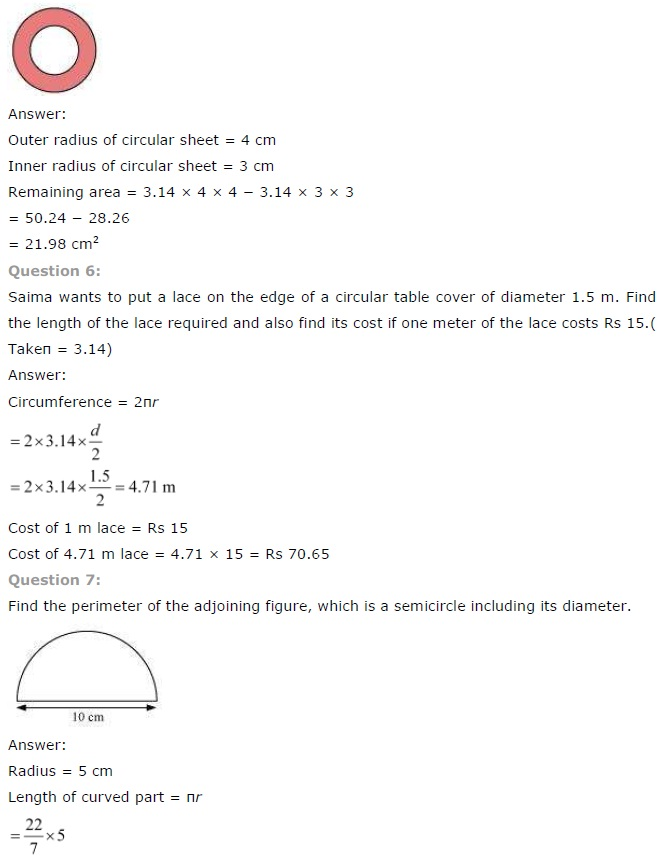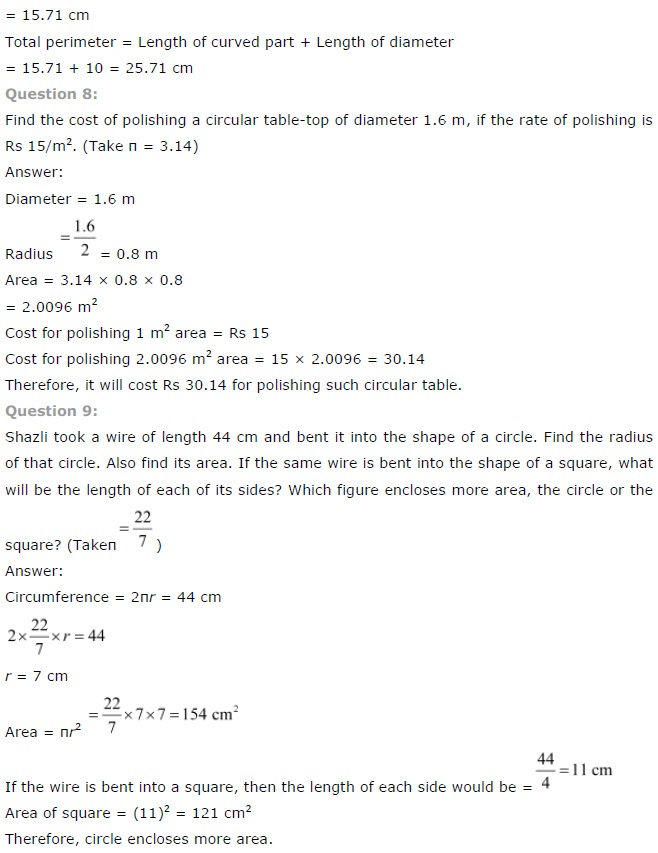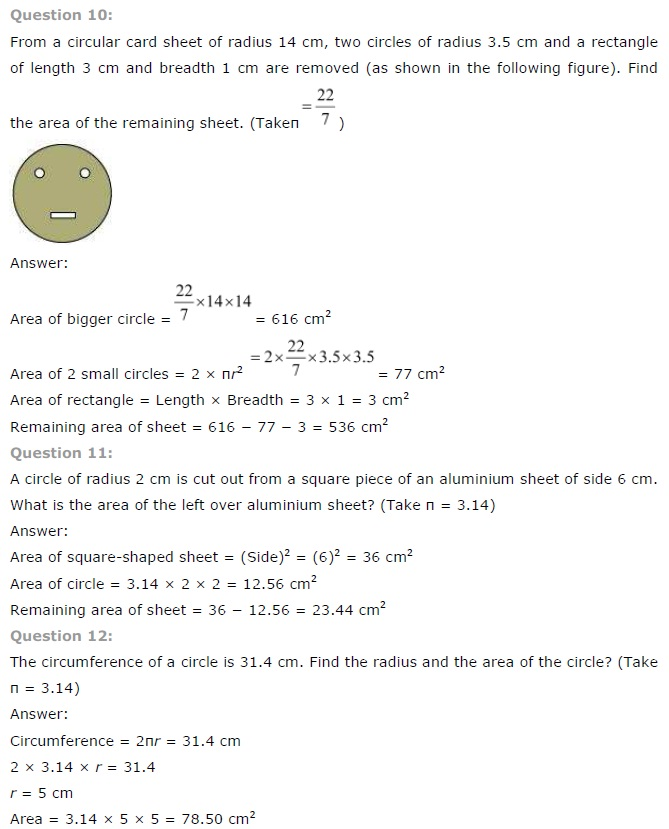+